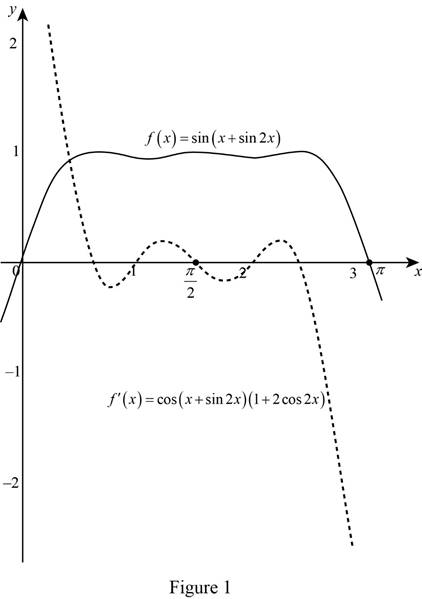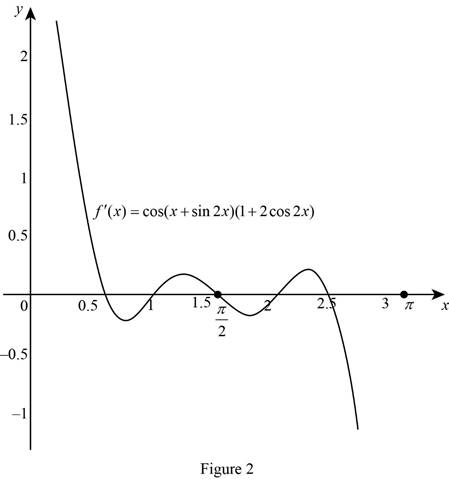# The slope of the function f ( x ) by using the graph of f ( x ) .### Single Variable Calculus: Concepts...

4th Edition
James Stewart
Publisher: Cengage Learning
ISBN: 9781337687805### Single Variable Calculus: Concepts...

4th Edition
James Stewart
Publisher: Cengage Learning
ISBN: 9781337687805

#### Solutions

Chapter 3.4, Problem 48E

(a)

To determine

## To sketch: The slope of the function f(x) by using the graph of f(x).

Expert Solution

### Explanation of Solution

The value of the derivative of the function at any point x can be estimated by drawing the tangent line at any point (x,f(x)) and then obtain the slope.

Mark the slope of the tangent as a point in y-axis and the value of x as a point in x-axis in the graph of f(x).

Proceed in the similar way at several points and obtain the rough graph of the f(x) as shown in Figure 1.

Graph:

The rough sketch of the derivative function, f(x) by using the graph of the function f(x) is shown below in Figure 1.(b)

To determine

### To calculate: The derivative of function f(x).

Expert Solution

The derivative of f(x)=sin(x+sin2x) is f(x)=cos(x+sin2x)(1+2cos2x)_.

### Explanation of Solution

Result used: Chain Rule

If h is differentiable at x and g is differentiable at h(x), then the composite function F=gh defined by F(x)=g(h(x)) is differentiable at x and F is given by the product,

F(x)=g(h(x))h(x) (1)

Calculation:

Obtain the derivative.

f(x)=ddx(f(x))=ddx(sin(x+sin2x))

Let h(x)=x+sin2x and g(u)=sinu  where u=h(x).

Apply the chain rule as shown in equation (1),

f(x)=g(h(x))h(x) (2)

The derivative of g(h(x)) is computed as follows,

g(h(x))=g(u)=ddu(g(u))=ddu(sinu)=cosu

Substitute u=x+sin2x in the above equation,

g(h(x))=cos(x+sin2x)

Thus, the derivative is g(h(x))=cos(x+sin2x).

The derivative of h(x) is computed as follows,

h(x)=ddx(x+sin2x)=ddx(x)+ddx(sin2x)=(1x11)+cos2x2=1+2cos2x

Thus the derivative is h(x)=1+2cos2x.

Substitute cos(x+sin2x) for g(h(x)) and 1+2cos2x for h(x) in equation (2),

F(x)=cos(x+sin2x)1+2cos2x=(1+2cos2x)cos(x+sin2x)

Therefore, the derivative of f(x)=sin(x+sin2x) is (1+2cos2x)cos(x+sin2x).

Graph:

Use the online graphing calculator to draw the graph of the derivative function f(x) as shown below in Figure 2.From Figure 2, it is observed that the given trigonometric function is differentiable everywhere and the rough sketch of the derivative f(x) in Figure 1 is same as the graph of the derivative f(x) in Figure 2.

### Have a homework question?

Subscribe to bartleby learn! Ask subject matter experts 30 homework questions each month. Plus, you’ll have access to millions of step-by-step textbook answers!